sklearn.datasets.make_classification¶

sklearn.datasets.make_classification(n_samples=100, n_features=20, n_informative=2, n_redundant=2, n_repeated=0, n_classes=2, n_clusters_per_class=2, weights=None, flip_y=0.01, class_sep=1.0, hypercube=True, shift=0.0, scale=1.0, shuffle=True, random_state=None)[source]

Generate a random n-class classification problem.

This initially creates clusters of points normally distributed (std=1) about vertices of an n_informative-dimensional hypercube with sides of length 2*class_sep and assigns an equal number of clusters to each class. It introduces interdependence between these features and adds various types of further noise to the data.

Without shuffling, X horizontally stacks features in the following order: the primary n_informative features, followed by n_redundant linear combinations of the informative features, followed by n_repeated duplicates, drawn randomly with replacement from the informative and redundant features. The remaining features are filled with random noise. Thus, without shuffling, all useful features are contained in the columns X[:, :n_informative + n_redundant + n_repeated].

Read more in the User Guide.

Parameters: n_samples : int, optional (default=100) The number of samples. n_features : int, optional (default=20) The total number of features. These comprise n_informative informative features, n_redundant redundant features, n_repeated duplicated features and n_features-n_informative-n_redundant-n_repeated useless features drawn at random. n_informative : int, optional (default=2) The number of informative features. Each class is composed of a number of gaussian clusters each located around the vertices of a hypercube in a subspace of dimension n_informative. For each cluster, informative features are drawn independently from N(0, 1) and then randomly linearly combined within each cluster in order to add covariance. The clusters are then placed on the vertices of the hypercube. n_redundant : int, optional (default=2) The number of redundant features. These features are generated as random linear combinations of the informative features. n_repeated : int, optional (default=0) The number of duplicated features, drawn randomly from the informative and the redundant features. n_classes : int, optional (default=2) The number of classes (or labels) of the classification problem. n_clusters_per_class : int, optional (default=2) The number of clusters per class. weights : list of floats or None (default=None) The proportions of samples assigned to each class. If None, then classes are balanced. Note that if len(weights) == n_classes - 1, then the last class weight is automatically inferred. More than n_samples samples may be returned if the sum of weights exceeds 1. flip_y : float, optional (default=0.01) The fraction of samples whose class are randomly exchanged. Larger values introduce noise in the labels and make the classification task harder. class_sep : float, optional (default=1.0) The factor multiplying the hypercube size. Larger values spread out the clusters/classes and make the classification task easier. hypercube : boolean, optional (default=True) If True, the clusters are put on the vertices of a hypercube. If False, the clusters are put on the vertices of a random polytope. shift : float, array of shape [n_features] or None, optional (default=0.0) Shift features by the specified value. If None, then features are shifted by a random value drawn in [-class_sep, class_sep]. scale : float, array of shape [n_features] or None, optional (default=1.0) Multiply features by the specified value. If None, then features are scaled by a random value drawn in [1, 100]. Note that scaling happens after shifting. shuffle : boolean, optional (default=True) Shuffle the samples and the features. random_state : int, RandomState instance or None (default) Determines random number generation for dataset creation. Pass an int for reproducible output across multiple function calls. See Glossary. X : array of shape [n_samples, n_features] The generated samples. y : array of shape [n_samples] The integer labels for class membership of each sample.

make_blobs
simplified variant
make_multilabel_classification

Notes

The algorithm is adapted from Guyon  and was designed to generate the “Madelon” dataset.

References

  I. Guyon, “Design of experiments for the NIPS 2003 variable selection benchmark”, 2003.

Examples using sklearn.datasets.make_classification¶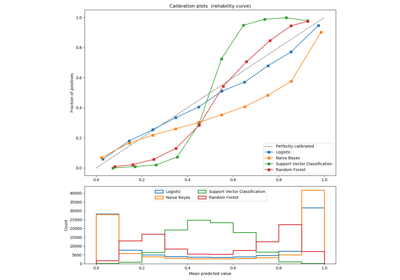Comparison of Calibration of Classifiers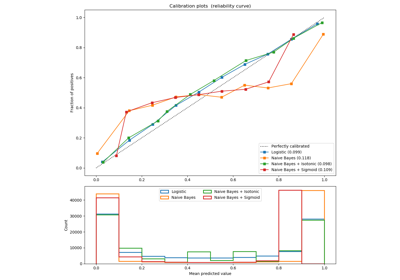Probability Calibration curves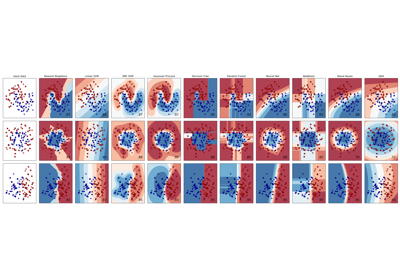Classifier comparison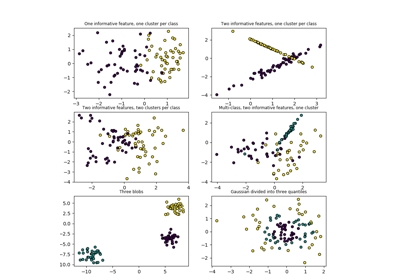Plot randomly generated classification dataset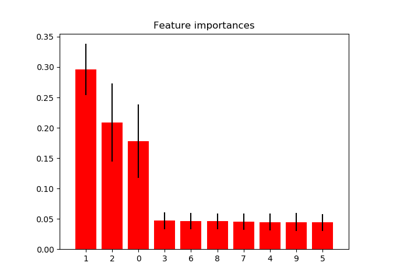Feature importances with forests of trees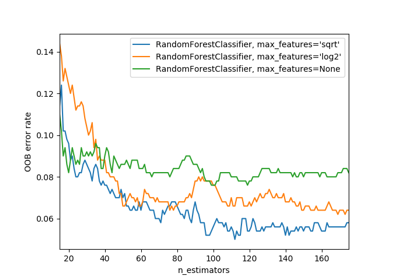OOB Errors for Random Forests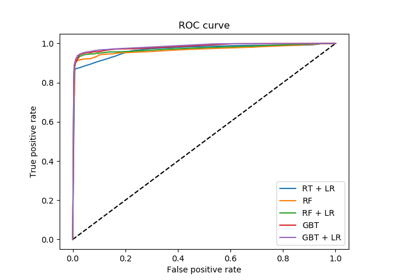Feature transformations with ensembles of treesPipeline Anova SVM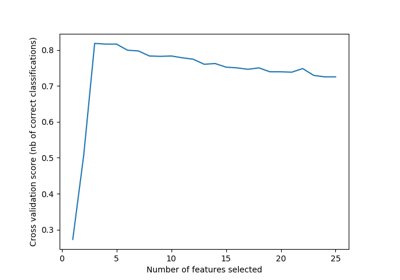Recursive feature elimination with cross-validation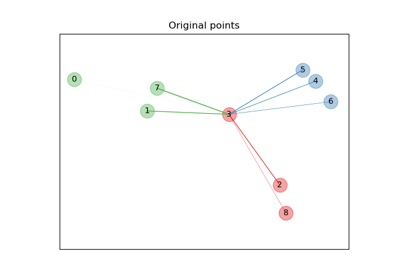Neighborhood Components Analysis Illustration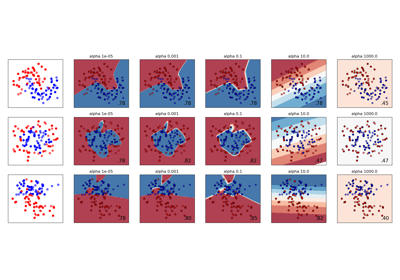Varying regularization in Multi-layer Perceptron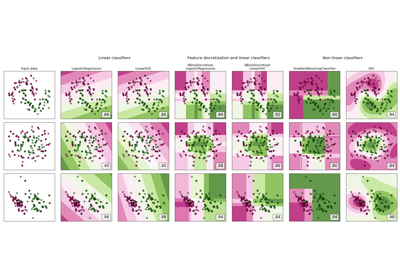Feature discretization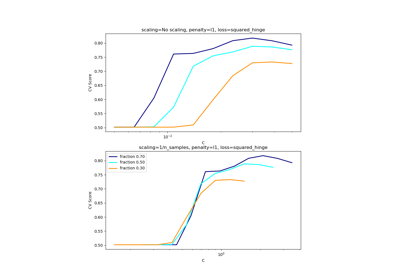Scaling the regularization parameter for SVCs